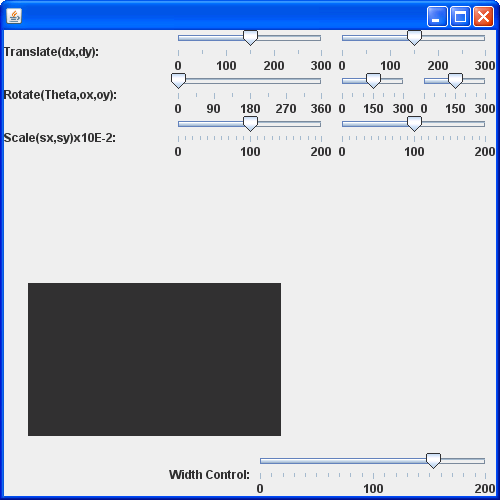# Compares two lines are returns true if they are equal or both null. : Line « 2D Graphics « Java Tutorial

```import java.awt.geom.Line2D;

public class Main {

/**
* Compares two lines are returns <code>true</code> if they are equal or
* both <code>null</code>.
*
* @param l1  the first line (<code>null</code> permitted).
* @param l2  the second line (<code>null</code> permitted).
*
* @return A boolean.
*/
public static boolean equal(final Line2D l1, final Line2D l2) {
if (l1 == null) {
return (l2 == null);
}
if (l2 == null) {
return false;
}
if (!l1.getP1().equals(l2.getP1())) {
return false;
}
if (!l1.getP2().equals(l2.getP2())) {
return false;
}
return true;
}

}
```
 16.12.Line 16.12.1. Draw a line16.12.2. draw Line from (10, 15) to (100, 115) 16.12.3. Line2D.Float16.12.4. Draw a point: use a drawLine() method 16.12.5. A line is drawn using two points 16.12.6. Dash style line 16.12.7. Line dashes style 2 16.12.8. Lines Dashes style 3 16.12.9. Line Dash Style 4 16.12.10. Draw Dashed 16.12.11. Compares two lines are returns true if they are equal or both null. 16.12.12. Creates a region surrounding a line segment by 'widening' the line segment.